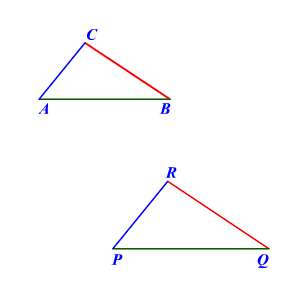# Side-Side-Side Similarity

If the corresponding sides of two triangles are proportional, then the two triangles are similar .If $\frac{AB}{PQ}=\frac{BC}{QR}=\frac{AC}{PR}$ ,

then triangles $\Delta ABC\text{\hspace{0.17em}}\text{\hspace{0.17em}}\text{and}\text{\hspace{0.17em}}\text{\hspace{0.17em}}\Delta PQR$ are similar.